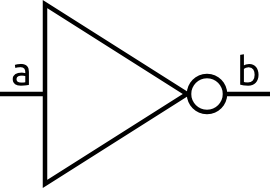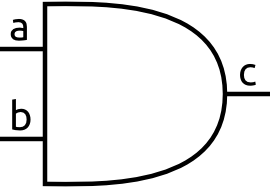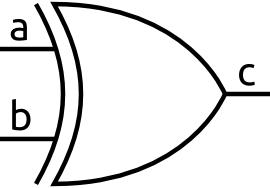# Digital Logic

What is digital logic? As you may or may not already know digital systems are based on two logic levels which are usually represented as 1's and 0's.

An interesting thing to note before we get too far in is that digital circuits are really made of the same stuff as any analog circuit. They have real voltages, with real currents, and those are never exact. The idea behind a digital system is that you simply design it so that there are two main stable states. That way even if you introduce noise it will remain close to that state. This makes digital circuits very robust and noise tolerant. It also allows up to abstract away what the actually voltages are and to just work with 0s and 1s.

So what is a 1? What is a 0? If you've ever worked with microcontroller, you know a lot of them run off 5V so we will use 5V in this example. If you were designing a system and it had an input that ranged from 0V to 5V what would you call a 0 or a 1? The most obvious answer is just split it in half. Anything 2.5V or less is a 0 and anything from 2.5V to 5V is a 1. That is what these circuits try to do, however, what happens when you are at 2.5V? Is that a 0 or a 1? Due to variations in the parts, temperature changes, power supply fluctuations, and an almost infinite number of causes it could end up being either!

Because of this uncertainty when between states we will call this region no man's land. Digital circuits are designed not to operate with voltages near this region. In fact digital circuits will have specifications that tell you the minimum voltage to be considered a 1 and the maximum voltage that will considered a 0 reliably. For now we won't worry about this since most of the time the circuits are designed to avoid this for us.

#### True or false?

Digital circuits are all based around these things called logic gates. Before we get into what the gates actually are, you need to know that they are all based on the idea that a 1 is true and that a 0 is false. This is known as boolean logic.

Digital logic circuits are often convenient to draw out with symbols. Each symbol represents some logic function that has at least one input and at least one output. In general the inputs are shown on the left and the outputs are shown on the right. That way the signals in the circuit flow from left to right.

#### Not

This is the symbol for a not gate.All that it does is output the opposite of a. So if a is true b is false. You can think of it's output as: is the input not true?

For example, if a is 1, then b wil output 0.

#### And

This is the symbol for an and gate.When a and b are both true, c is true. If either a or b is false then c will be false. You can think of it's output as: is input a and input b true?

For example, if a is 1 and b is 0, then the output, c, will be 0. That is because both inputs are not true, so the output is false.

#### Or

This is the symbol for an or gate.The output of this gate, c, is true when either a or b are true. You can think of it's output as: is input a or input b true?

For example, if a is 1 and b is 0, then the output, c, will be 1. That is because at least one of the inputs is true, so the output is true.

#### Xor

This is the symbol for an xor gate.This gate you usually don't see as often as the other three, but it is none the less important. This gate is true only when either a or b is true but not when a and b are true. It is an exclusive or. You can think about it as: is input a different from input b?

Another useful way to look at xor gates it to think about that output as: is there an odd number of 1s? This way of thinking about it is useful for xor gates that have more than two inputs.

For example, if a is 1 and b is 1, then the output, c, will be 0. That is because the inputs are the same so the output is false, or because there are an even number of 1s so the output is false.

#### Variations

These are only the basic types of these gates. There are many variations of them. The most common variations you will see are combinations of, and, or, xor, with a not gate at the output. Those gates are called nand, nor, and xnor respectively. They are represented the same as their base gate but they have a circle on the output, just like the not gate does.

The circle represents an inversion (a not gate) and they don't only have to be on the output. They can be on any of the inputs as well. It is just a compact way to draw the schematic so you don't have lots of not gates everywhere.